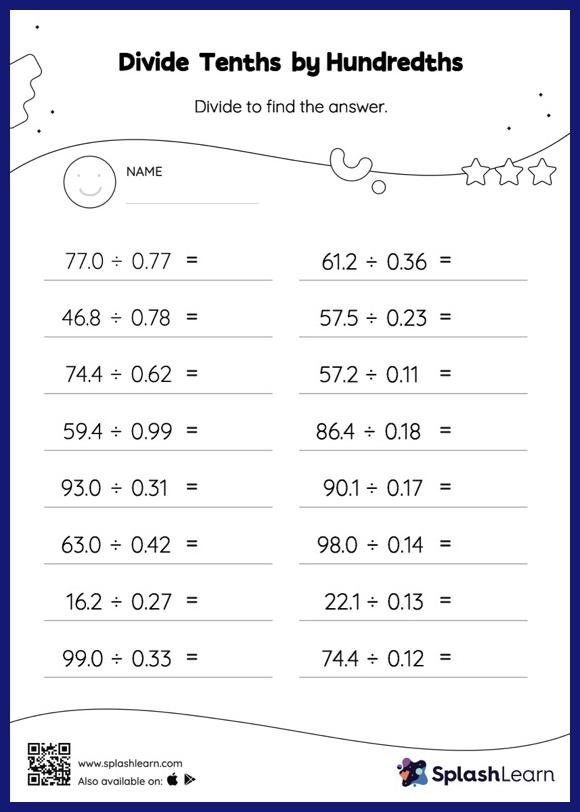# Divide Tenths by Hundredths without Remainder: Horizontal Division Worksheet

Home > Divide Tenths by Hundredths without Remainder: Horizontal DivisionChildren use their math skills to complete this divide tenths by hundredths without remainder worksheet. Students first divide as though there is no decimal when dividing two decimals. They then add a decimal point at the correct location in the quotient depending on the number of decimal digits in each number. This divide tenths by hundredths without remainder worksheet gives students a thorough practice of this concept.